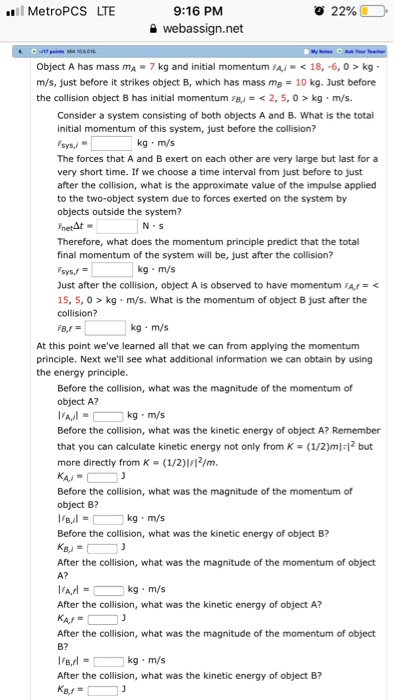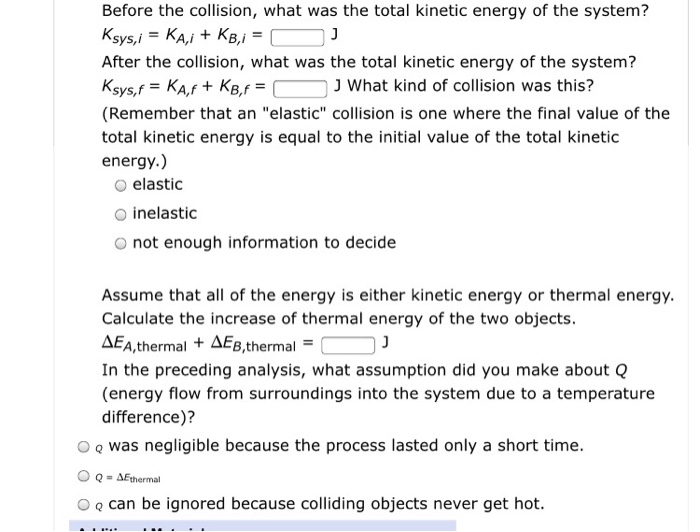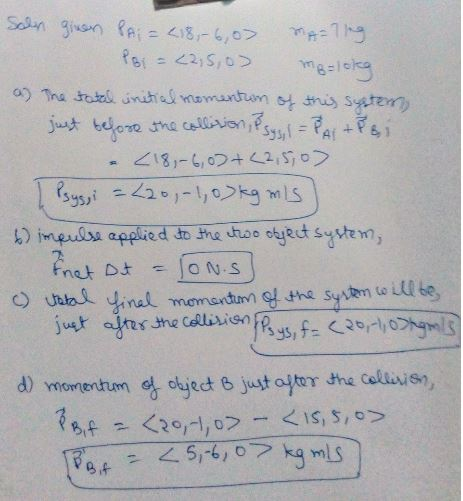# MetroPCS LTE 9:16 PM 9 webassign.net Object A has mass mA-7 kg and initial momentum A<18,-6, 0> kg m/s, just before it strikes object B, which has mass mB 10 kg. Just before the collision...MetroPCS LTE 9:16 PM 9 webassign.net Object A has mass mA-7 kg and initial momentum A kg m/s, just before it strikes object B, which has mass mB 10 kg. Just before the collision object B has initial momentum B 2, 5, 0> kg m/s. Consider a system consisting of both objects A and B. What is the total initial momentum of this system, just before the collision? lys,- The forces that A and B exert on each other are very large but last for a very short time. If we choose a time interval from just before to just after the collision, what is the approximate value of the impulse applied to the two-object system due to forces exerted on the system by objects outside the system? netAt Therefore, what does the momentum principle predict that the total final momentum of the system will be, just after the collision? sys,f Just after the collision, object A is observed to have momentum FAr kg m/s. What is the momentum of object B just after the collision? kg m/s N S At this point we've learned all that we can from applying the momentum principle. Next we'll see what additional information we can obtain by using the energy principle. Before the collision, what was the magnitude of the momentum of object A? kg m/s Before the collision, what was the kinetic energy of object A? Remember that you can calculate kinetic energy not only from K-(1/2)m 12 but more directly from K (1/2)I2/m Before the collision, what was the magnitude of the momentum of object B? kg m/s Before the collision, what was the kinetic energy of object B? After the collision, what was the magnitude of the momentum of object A? kg m/s After the collision, what was the kinetic energy of object A? After the collision, what was the magnitude of the momentum of object B? kg m/s After the collision, what was the kinetic energy of object B?
Before the collision, what was the total kinetic energy of the system? After the collision, what was the total kinetic energy of the system? J What kind of collision was this? (Remember that an "elastic" collision is one where the final value of the total kinetic energy is equal to the initial value of the total kinetic energy.) O elastic O inelastic O not enough information to decide Assume that all of the energy is either kinetic energy or thermal energy. Calculate the increase of thermal energy of the two objects ΔΕΑ,thermal + ΔΕΒ,thermal- In the preceding analysis, what assumption did you make about Q (energy flow from surroundings into the system due to a temperature difference)? O o was negligible because the process lasted only a short time. O o can be ignored because colliding objects never get hot.

according to the chegg rule we have to answer only four sub parts at a time.##### Add Answer of: MetroPCS LTE 9:16 PM 9 webassign.net Object A has mass mA-7 kg and initial momentum A<18,-6, 0> kg m/s, just before it strikes object B, which has mass mB 10 kg. Just before the collision...
More Homework Help Questions Additional questions in this topic.